# output-devices

this is week 2 page.

#### Group assignment:

• Measure the power consumption of an output device
• Document your work (in a group or individually)

#### Individual assignment:

• Add an output device to a microcontroller board you’ve designed and program it to do something

#### lets run the programmer i will use Arduino as ISP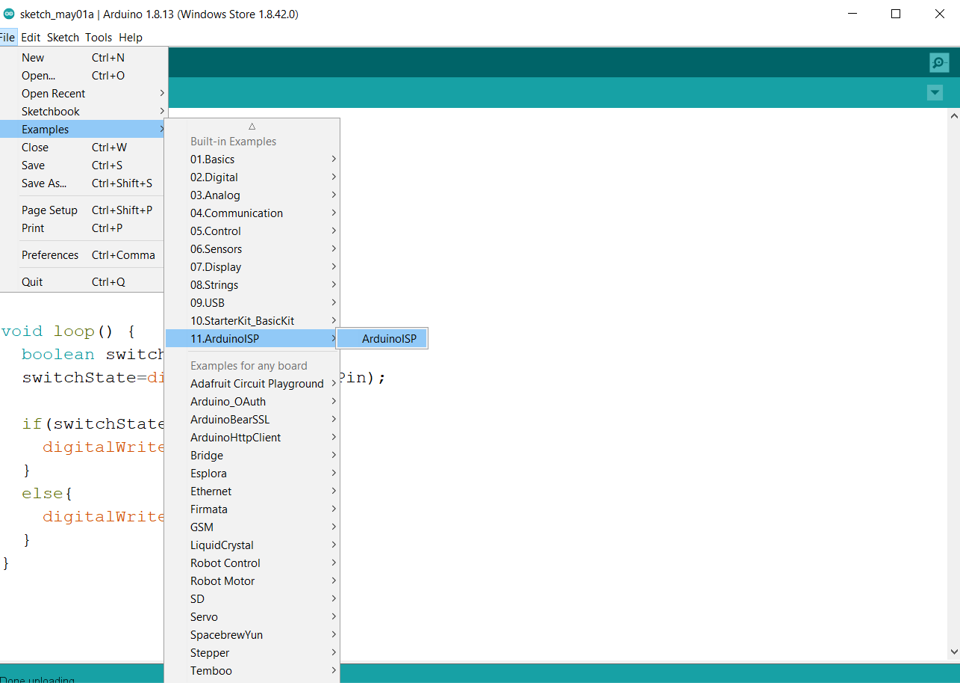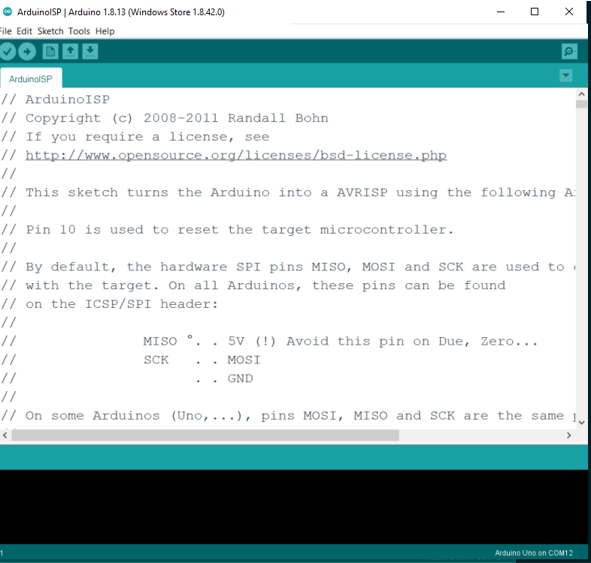#### connect isp with arduino

• Connect pin 13 from Arduino = pin 7 in Attiny 45 = SCK ISP
• Connect pin 12 from Arduino = pin 6 in Attiny 45 = MISO ISP
• Connect pin 11 from Arduino = pin 5 in Attiny 45 = MOSI ISP
• Connect pin 10 from Arduino = pin 1 in Attiny 45 = RESET ISP
• Connect pin 5v from Arduino = pin 8 in Attiny 45 = VCC ISP
• Connect pin GND from Arduino = pin 4 in Attiny 45 = GND ISP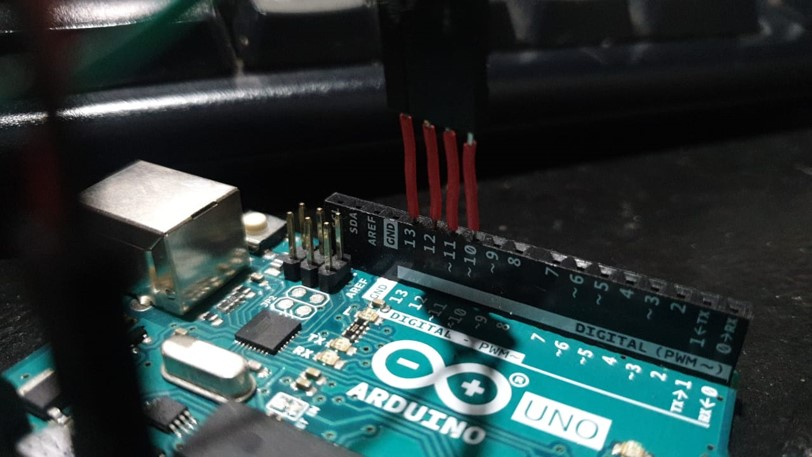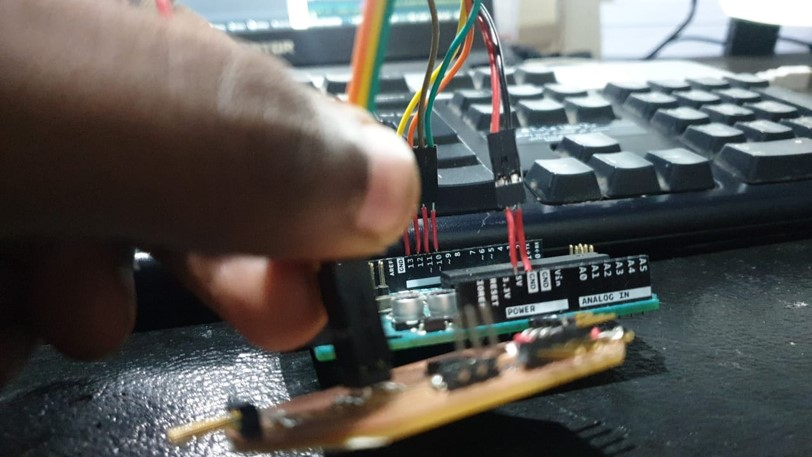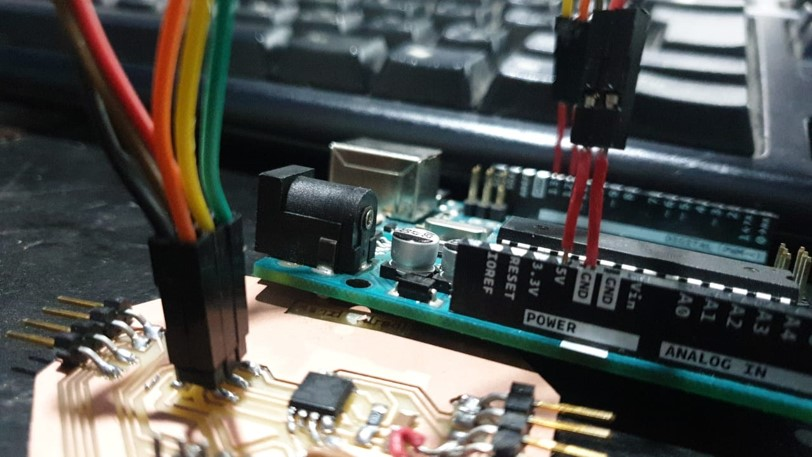if you dont know how to upload the program follow my steps that i have explained in Embedded Programming upload the first example

#### PWM

Pulse Width Modulation(PWM) is a digital technology that uses the amount of power delivered to a device that can be changed. It generates analogue signals by using a digital source. A PWM signal is basically a square wave which is switched between on and off state. The duty cycle and frequency of a PWM signal determine its behaviour.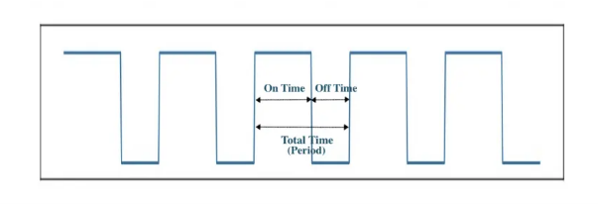#### Duty Cycle in PWM

The duty cycle of the PWM signal refers to the ratio of the time that the signal is in a high(on) state over the total time it takes to complete one cycle. It is commonly expressed as a percentage or a ratio.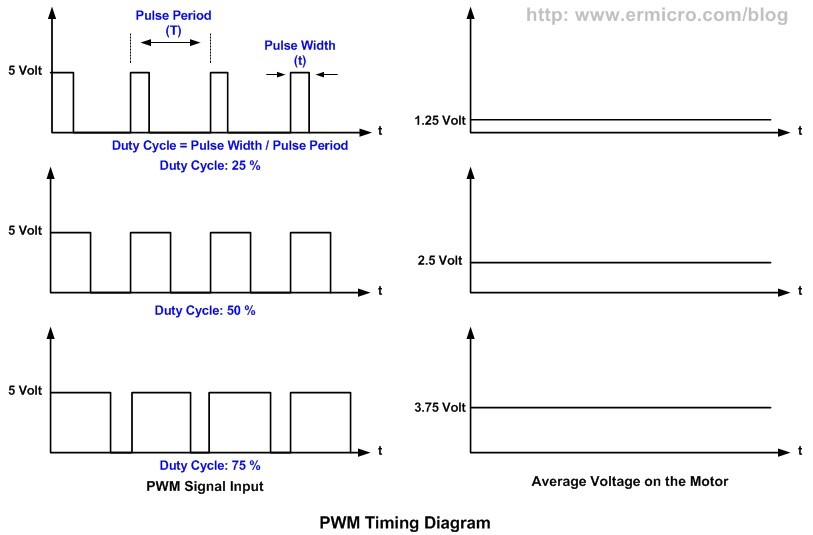A 50% duty cycle means that the high state takes half of the time and the low state takes the other half of the time, this is the same as an ideal square wave. If this ratio is greater than 50%, the logic high signal takes a longer time than logic low, vice versa. Thus, a 100% duty cycle means the signal is always on(full-scale), and the 0% duty cycle means the signal is always off(grounding).

#### Frequency of PWM signal

A period is equal to the time this signal completes a one-and-off cycle. The frequency is the number of times a periodic change is completed per unit time and it is the inverse of the period. It determines the speed at which the PWM completes one cycle, which means the speed at which the signal switches between high and low states. If we turn the digital signal on and off repeatedly with a high enough frequency, the output will behave like an analogue signal with a constant voltage.

##### applying Pulse Width Modulation(PWM)

one of the famous example of applying PWM with Arduino is the Fade Example, you can find it built in on Arduino Ide software, Go To File –» Example –» Basic –» Fade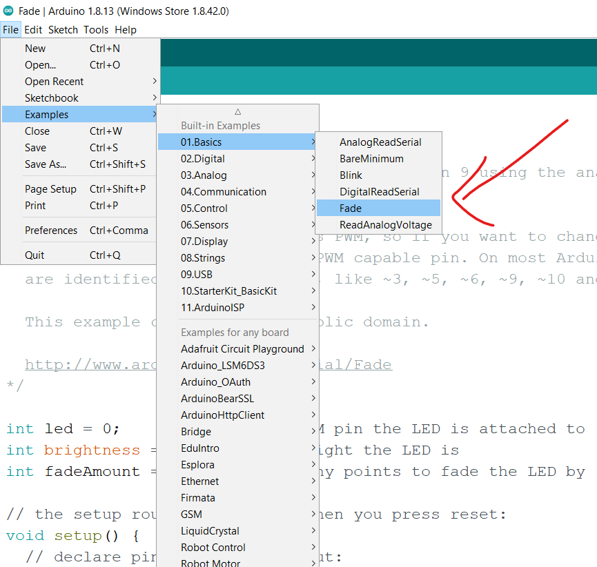• the only modification to make the example run in Attiny45 is to change the led pin

• number to be realtive to attiny PWM pins

According to the Datasheet (page 27) that Hardware Pwm can be run in PB4-PB0-PB1

#### Pin Configurations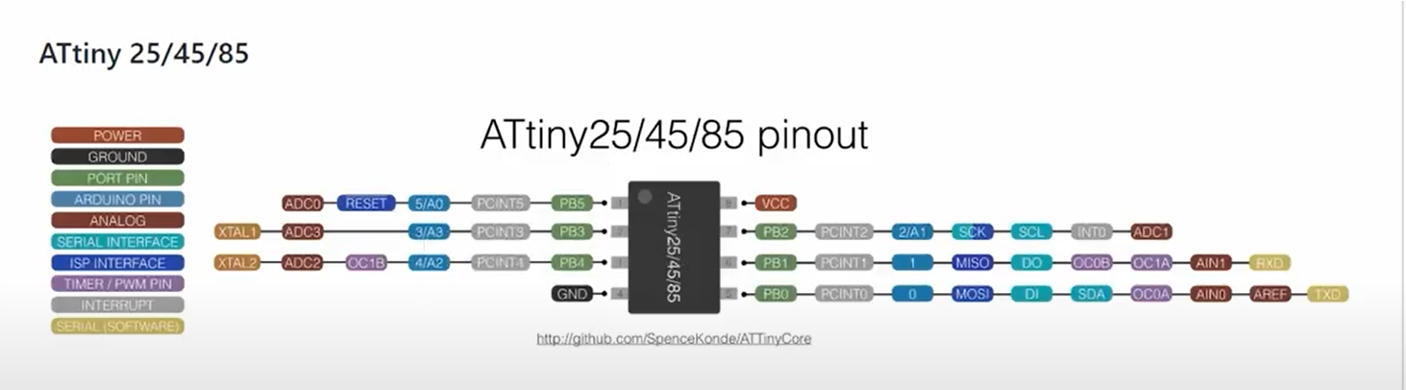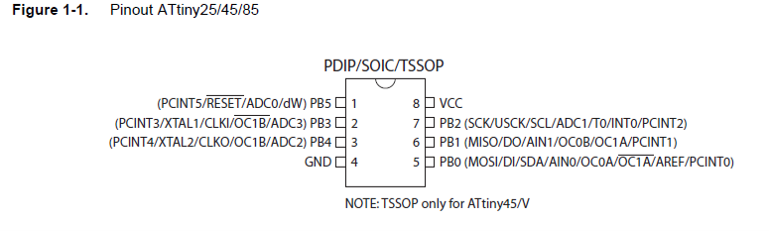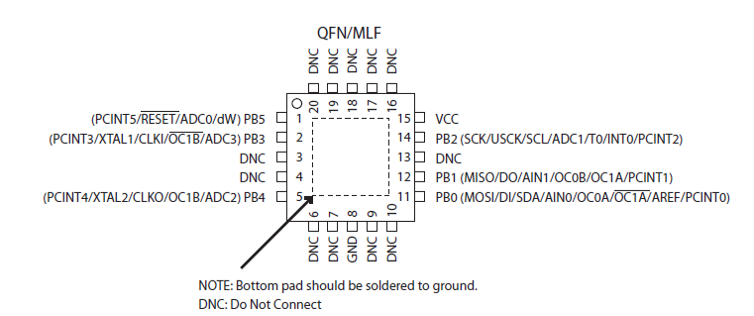so change the led pin to one of theses, i will choose 0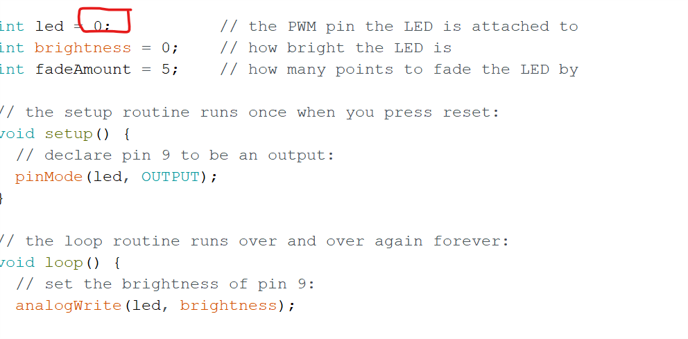• and dont forget to change the board that you are using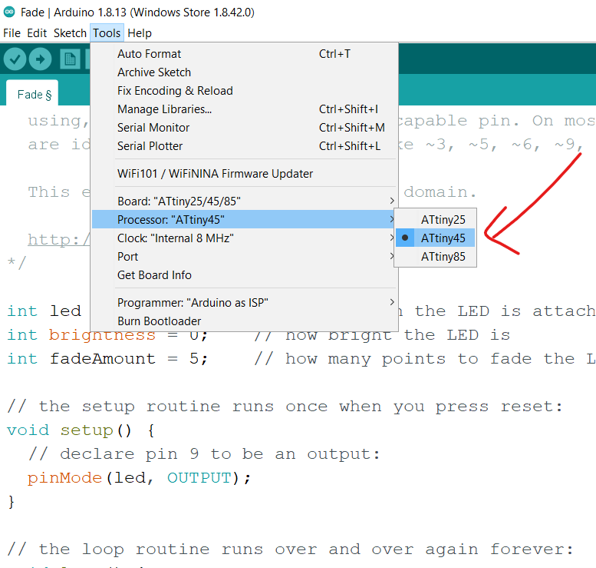#### Lets understanf the Code first then we can run it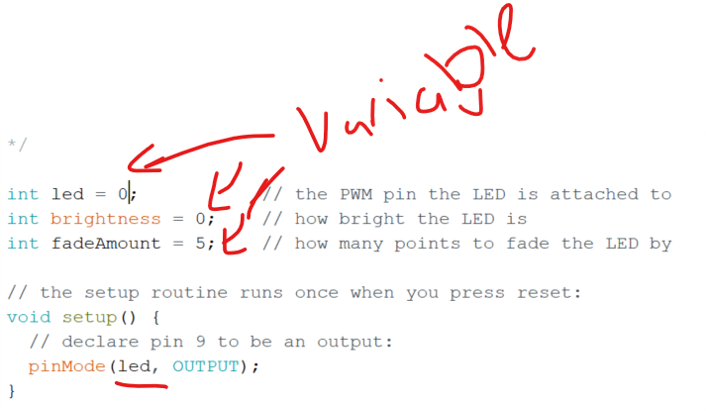by understanding that arduino ide is running the code from top to bottom its smart to think as excuter and understand each line only when it time to do action with it.

so at the start we have three variables that have values but it not make sense yet so we have the variables as

• " led " that have the value = " 0 "
• " brightness " that have the value = " 0 "
• " FadeAmount " that have the value = " 5 "
###### any code will be written in the void setup will be executed only one time

we have pinMode (led,Output); , now we now that the variable led is used here to define the functionality of a pin, this line means that pin 0 will be used as output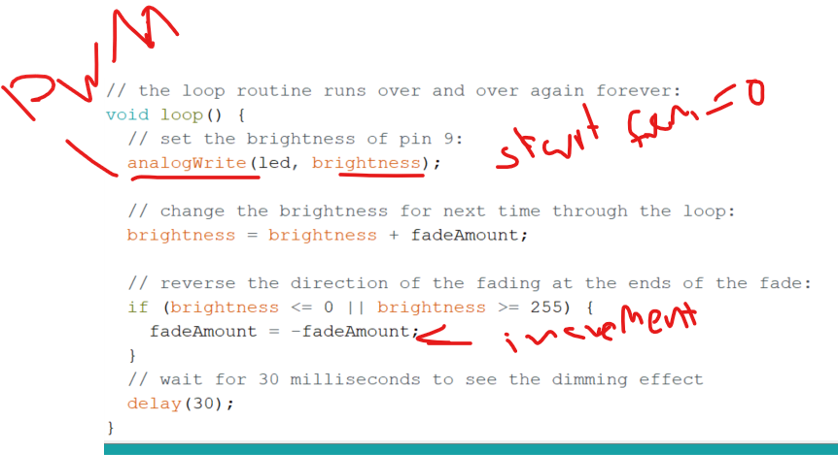###### any code will be written in the void setup will be executed Forever as long as arduino have power

`analogWrite (led,brightness);` analogwrite is the way to tell the arduino ide that you need to generate PWM signal, and here now we know that pin 0 will be used to generate a pwm signal with dutycyle = brightness , and brightness is the variable that contain the value of 0 at the start of the program

` brightness = brightness + fadeAmount;` this line will update the value that stored in the variable brightness with amount of “fadeAmount”, and we know that it contain the value “5”

so that’s mean each time the loop come to this line will add 5 and so on

`if (brightness <= 0 || brightness >= 255) { fadeAmount = -fadeAmount;}` here is a Boolean condition that will run only if the condition is true

and if the condition was true it will execute the code between its brackets, and what’s inside the brackets is `fadeAmount = -fadeAmount ;` that will change the value of the fadeamount to be either negative or positive by multiplying the value with -1

so here we understand that the led will start from the lowest dutycyle = 0 which = 0V to the highst dutycyle 255 which = 5V

#### Servo

A Servo Motor is a small device that has an output shaft. This shaft can be positioned to specific angular positions by sending the servo a `Pulse Width Modulation(PWM)` coded signal.

As long as the coded signal exists on the input line, the servo will maintain the angular position of the shaft. If the coded signal changes, the angular position of the shaft changes.

In practice, servos are used in radio-controlled airplanes to position control surfaces like the elevators and rudders. They are also used in radio-controlled cars, puppets, and of course, robots.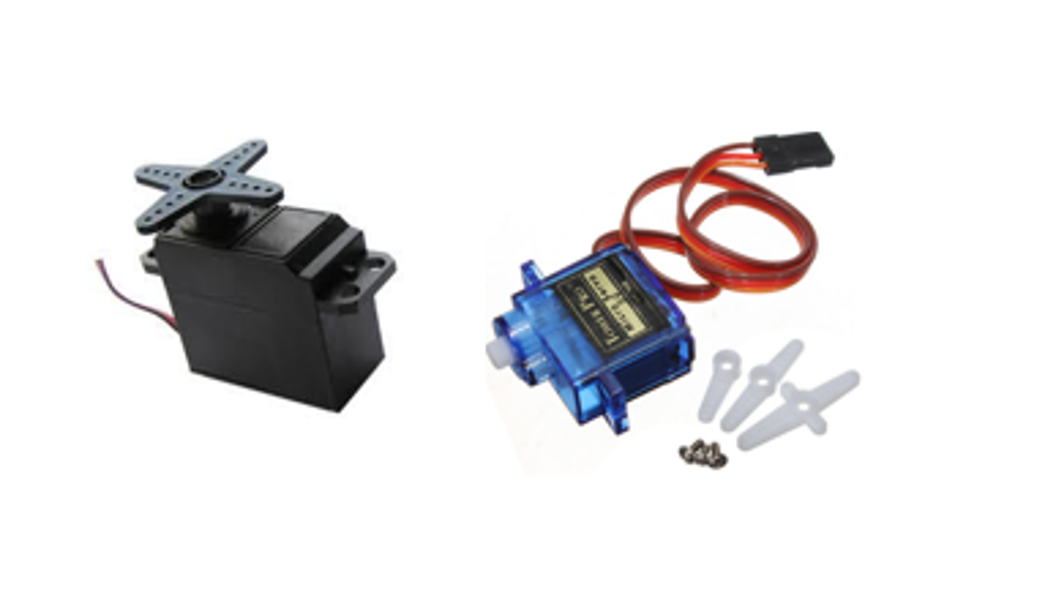#### whats inside the servo ?

Servo is a general term for a closed loop control system.

A closed loop system uses the feedback signal to adjust the speed and direction of the motor to achieve the desired result.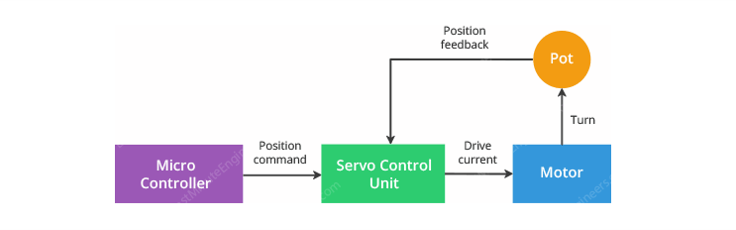RC servo motor works on the same principal. It contains a small DC motor connected to the output shaft through the gears.

The output shaft drives a servo arm and is also connected to a potentiometer (pot).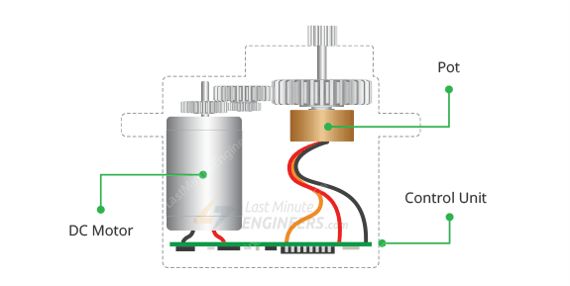The potentiometer provides position feedback to the servo control unit where the current position of the motor is compared to the target position.

According to the error, the control unit corrects the actual position of the motor so that it matches the target position.

#### How to control Servo Motors with PWM ?

You can control the servo motor by sending a series of pulses to the signal line. A conventional analog servo motor expects to receive a pulse roughly every 20 milliseconds (i.e. signal should be 50Hz).

The length of the pulse determines the position of the servo motor.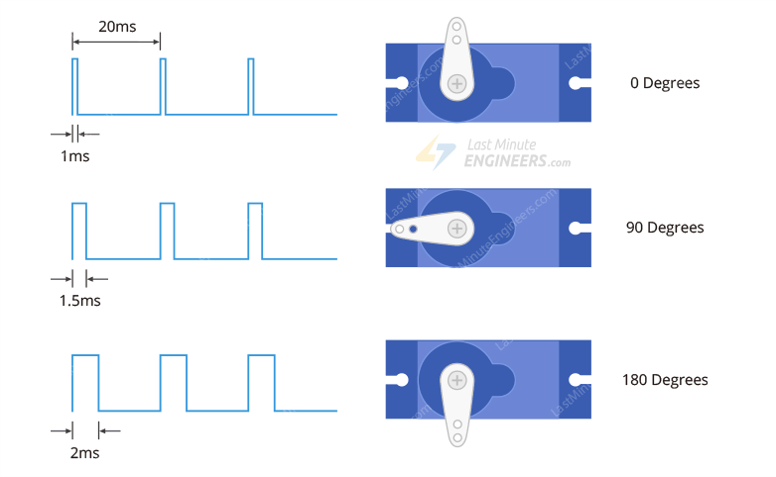If the pulse is high for 1ms, then the servo angle will be zero. If the pulse is high for 1.5ms, then the servo will be at its center position. If the pulse is high for 2ms, then the servo will at 180 degrees. Pulses ranging between 1ms and 2ms will move the servo shaft through the full 180 degrees of its travel.

#### Connection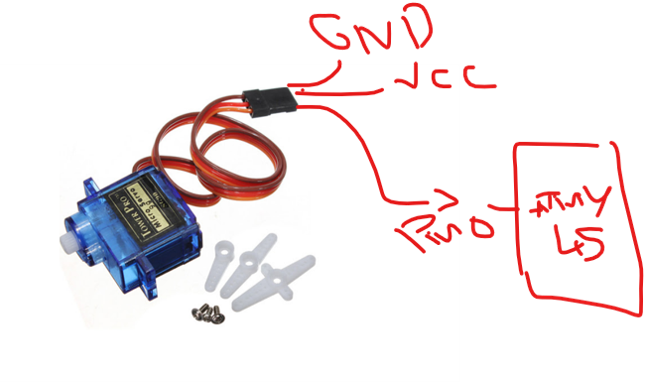#### Programming

i used to test each servo with arduino by running the ready example " Sweep "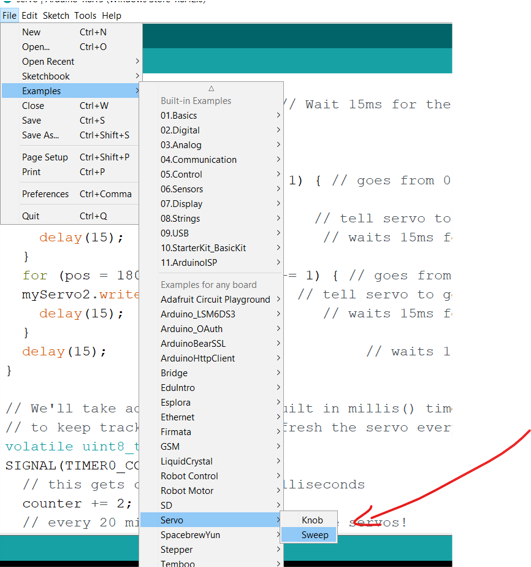but each time i upload the code it give me an error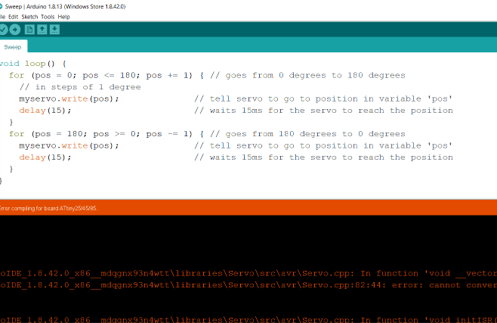``````servo volatile uint8_t* {aka volatile unsigned char*}' to 'volatile uint16_t* {aka volatile unsigned int*}' for argument '2' to 'void handle_interrupts(timer16_Sequence_t, volatile uint16_t*, volatile uint16_t*)' handle_interrupts(_timer1, &TCNT1, &OCR1A); ^

``````
###### The normal Servo.h library does not support the attiny85 (or the tiny861) because these have a very different timer1.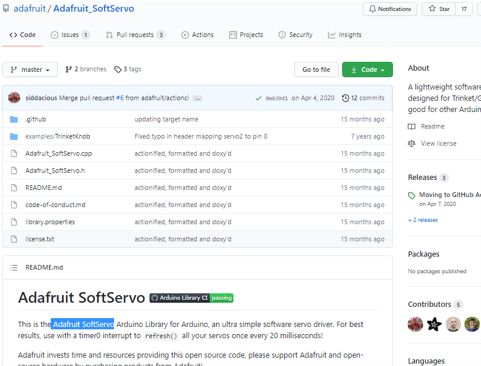you can have it from here

#### TikerKonb example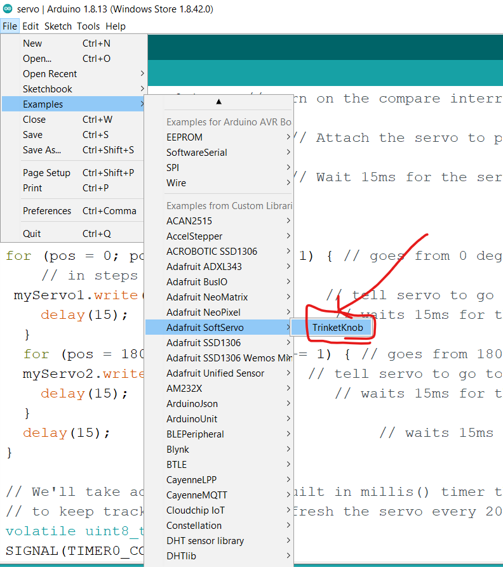Starting from this example i made the modification that i need , and choosed the pin from the my board to test it on it , and i mixed the code with the sweep example to make it do the same function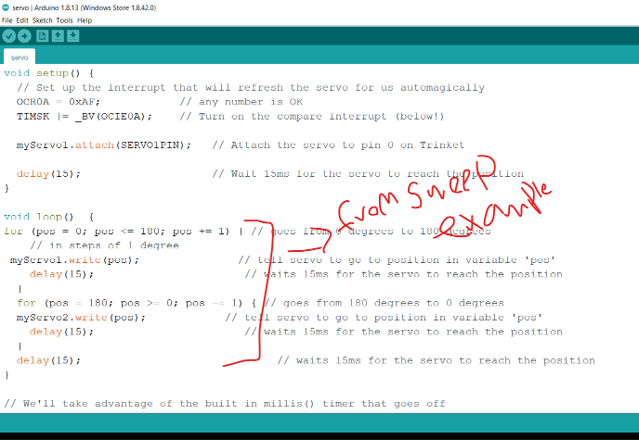#### here is it on Action

The WS2812B Addressable LED Strip is an intelligent light source that contains a control IC and an RGB LED in a same package, usually in SMD 5050 form factor. The following image shows a strip of WS2812B LEDs.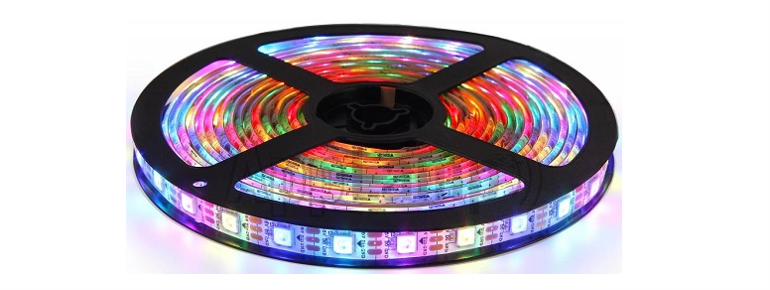Individual WS2812B LED Block has four pins namely VDD, VSS (GND), DIN and DOUT. The functions of these pins are very simple and the following table gives a simple function description of the pins.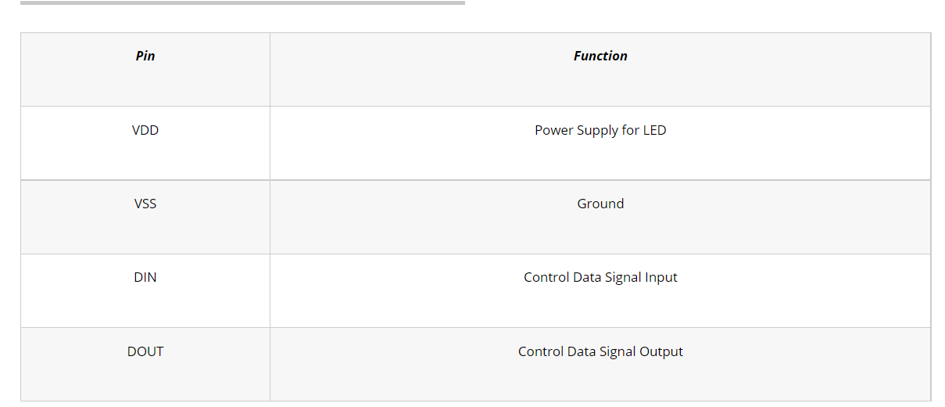To understand how the WS2812B LEDs can be individually controlled, the following application circuit will be useful. All the WS2812B LEDs are connected in a cascade manner where the DO of the first LED is connected to the DIN of the second LED and so on.

But the first LED has to receive data through its DIN pin from a Microcontroller like Arduino. The following image shows the typical application circuit block diagram.The data transfer protocol used by the WS2812B LEDs is NRZ Mode. The first DIN Port of the WS2812B LED array receives data from the microcontroller. The data for individual pixel is of 24-bit that consists of individual Red, Green and Blue LED control data of 8-bits each. The order of data must be GRB and the composition of the 24-bit data is shown below. Note that HIGH bit data is sent first.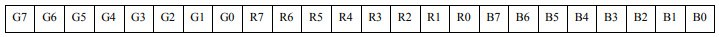Once the first WS2812B Block receives the first 24-bit data, the data is sent to its internal latch for further decoding. The remaining data is reshaped by its signal reshaping and amplification circuit and is passed to the next pixel in the cascade through the DO pin.

#### Connection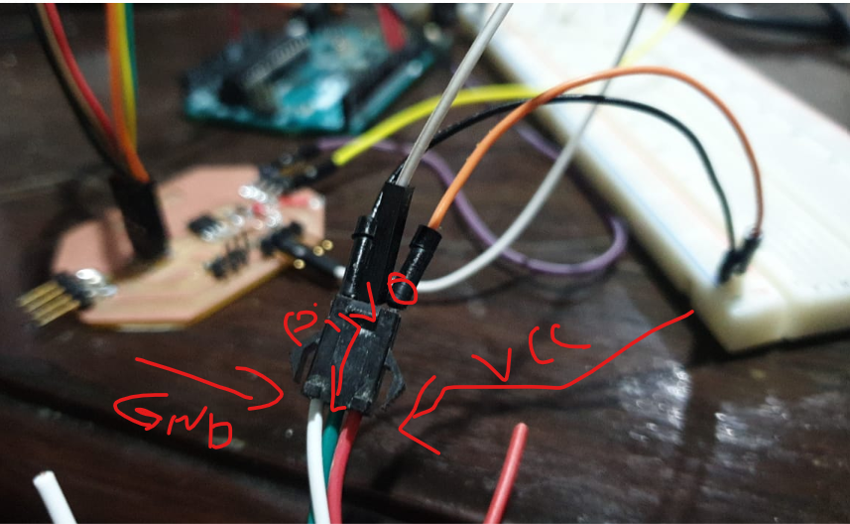LED —- Attiny45

Red —- »> 12 V White —- »> GND Green —- »> pb0
GND —- »> GND

Make sure to connect the gnd to together so the signal will stable

i used the liberrary FastLED to sontrol the strip light

#### Problem

the first problem was that the memory was not enough in the attiny45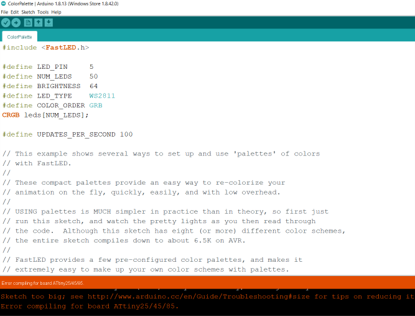• second problem was that all leds on the strip gets light up during the code uplad, and they never turn off even if i write for them color black
• and the code is not responding

#### Solved

to solve these issue

• i reduced the number of leds to be controlled
• i cut the rest that is not in the program

#### Attiny85

i found that Attiny85 has more memry than attiny45 so i gave it a test to see if it can drive more leds